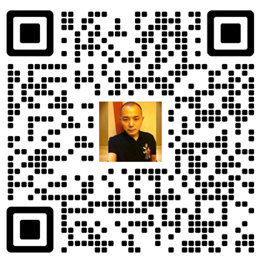﻿ 2021年山东成人高考高起点数学(文科)真题及答案-滕州成人高考_滕州成考「包通过」滕州成考报名_滕州函授报名中心
欢迎访问滕州成考网！ 本网站为成考报名咨询，主要为广大考生提供报成考指导服务，网站信息仅供学习交流使用，官方信息以山东省教育招生考试院www.sdzk.cn为准。
• 咨询、投诉电话：15562253555

# 2021年山东成人高考高起点数学(文科)真题及答案

2020-03-18 21:27   来源：成人高考报名 学习交流群+ 加入微信公众号+ 关注2021年成人高等学校招生全国统一考试

数学(文科)

本试卷分第I卷(选择题)和第II卷(非选择题)两部分,共4页。满分150分考试时间120分钟。

第I卷(选择题，共85分)

一、选择题:本大题共17小题，每小题5分，共85分。在每个小题给出的四个选项中，选出一项符合题目要求的。

1.设全集U=(1,2,3,4},集合M=(3.4],则CJM =

A.{2,3}B.[2,4}C{L4}D.(1.2}

[答案] D

[解析]求补集，是集合缺少的部分，应该选D2.函数y = cos4x的最小正周期为

A.π/4

B.π/2

C.π

D.π

[答案] B

3.设甲: b=0;乙:函数y= kx + b的图像经过坐标原点，则

A.甲是乙的充分条件但不是必要条件

B.甲是乙的必要条件但不是充分条件

C.甲是乙的充要条件

D.甲既不是乙的充分条件也不是乙的必要条件[答案] C

[解析]本题考查了充分条件和必要条件的知识点，

5.函数y= 根号1- x石的定义域是

A. {x|x≥-1}

B. {x|x≤ 1}

C. {x|x≤-1}

D. {xI-1≤x≤1}

[答案] D

[解析] 1-x°≥0时，原函数有意义，即x°≤1即{x|-1≤x≤1}

6.设0

A.1<2*x<2

B. 0<2*< 1

C. log1/2x< 0

D. log:x > 0

[答案] A

7.不等式|x+1/2|>1/2的解集为

A. {x|-1

B. {x|x>0或x<-1}

C. {x|x>-1}

D. {x|x< 0}

[答案] B

8.甲、乙、丙、可4人排成一行，其中甲、乙必须排在两端，则不同的排法共有

A.2种B.4种C.8种D，24种

[答案] B

9.若向量a=(1,1), b=(1,-1)，则≌a-gb=A. (-1,2) B. (1,-2)C. (1,2)

D. (-1,-2)

[答案] A

10.log21 + 161/2+(-2)°=

A.5B.4C.3D.2

[答案] B

[解析] logg1+ 161+(-2)°=0+4+1=5

11.函数y=x≥- 4x- 5的图像与x轴交于A、B两点，则|AB|=

A.3B.4C.5D.6

[答案] D

[解析] x好-4x-5= 0解得x=-1或x= 5,则A、B两点距离lABI= 612.下列函数为奇函數的是A. y=-2x+3B. y=-EC. y=x2-3D. y= 3cosx[答案] B

[解析]满足f(-x)=-f6)为奇函数

13.双曲线”-些= 1的焦点坐标为

A. (-5,0)，。 (5,0)

B. (-V70)，(V70)

C. (0,-5)，60,5)

D. (0，一7)，(0,V7)

[答案] A

[解析]显然x2的系数大于0，则焦点在x轴。又c=√a°+b=V9+ 16= 5,则焦点坐标为(-5,0)，(5,0)

以下题目缺少题干，答案仅供参考14.B15.B .16.A .17.C

二、填空题:本大题共4小题，每小题4分，共16分。#### 【可加下方微信免费报名】

【微信15562253555 姚老师】 【请广大考生提前备考】
##### 加入山东成考公众号山东成考网

(扫一扫加入)
##### 加入山东成考交流群﻿